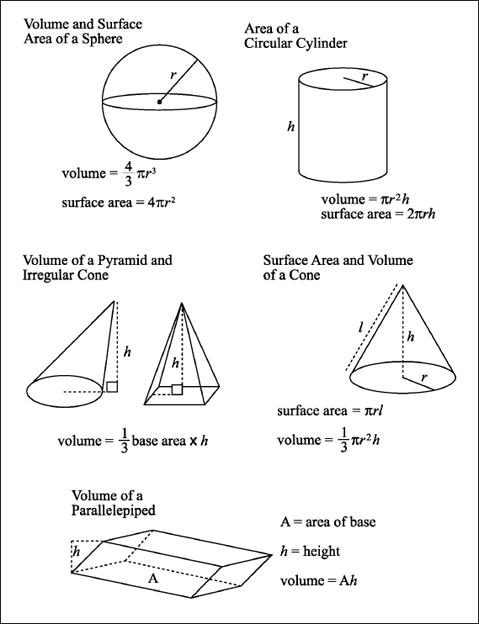NextPrevious

# What are the common mathematical formulas for area?

Area of a rectangle:

Area = length times width

A = lw

Area = altitude times base

A = ab

Area of a circle:

Area = pi times the radius squared

A = pr2 or A = 1/4pd2

Area of a triangle:

Area = one half the altitude times the base A = 1/2ab

Area of the surface of a sphere:

Area = four times pi times the radius squared A = 4pr2 or A = pd2

Area of a square:

Area = length times width, or length of one side squared A = s2

Area of a cube:

Area = square of the length of one side times 6

A = 6s2

Area of an ellipse:

Area = long diameter times short diameter times 0.7854.

What are the common mathematical formulas for volume?

Volume of a sphere:

Volume = 4/3 times pi times the cube of the radius

V = 4/3 × pr3

Volume of a pyramid:

Volume = 1/3 times the area of the base times the height

V = 1/3bh

Volume of a cylinder:

Volume = area of the base times the height

V = bh

Volume of a circular cylinder (with circular base):

Volume = pi times the square of the radius of the base times the height

V = pr2h Volume of a cube:

Volume = the length of one side cubed

V = S3

Volume of a cone:

Volume = 1/3 times pi times the square of the radius of the base times the height

V = 1/3 pr2h

Volume of a rectangular solid:

Volume = length times width times height

V = lwhClose

This is a web preview of the "The Handy Science Answer Book" app. Many features only work on your mobile device. If you like what you see, we hope you will consider buying. Get the App